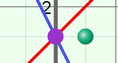# Solving Linear Systems (Slope-Intercept Form)

### DESCRIPTION

Solve systems of linear equations, given in slope-intercept form, both graphically and algebraically. Use a draggable green point to examine what it means for an (x, y) point to be a solution of one equation, or of a system of two equations.

Full Lesson Info